Proudly American#### Got questions?

Email us: [email protected]

# Learn How To Use Excel Round Down Function, the Easy Way!The ROUNDDOWN formula in Microsoft Excel is the subject of this article. The function returns a number that has rounded down to a specified number of decimal places. Its unique qualities are what make it helpful. This function differs from ordinary rounding in that it decreases the value of all numbers.

For example, only numbers below five are rounded down in standard rounding, whereas values greater than five are round-up. All numbers are always rounded down when using ROUNDDOWN. It could be handy in a variety of situations.

The ROUNDDOWN function in Excel returns a number that has round-down to a specified number of decimal places. Unlike normal rounding, which only rounds down values less than 5, ROUNDDOWN rounds down all numbers. The ROUNDDOWN function in Microsoft Excel describes in this article, along with its formula syntax and usage.

Learn how to round down quickly and easily in Excel. Excel’s ROUND DOWN function ensures that data is always rounded-down to the specified number of decimal places. We’ll look at how to use this feature efficiently as a group.

## What is the ROUNDDOWN formula in Excel?

### Description

This formula rounds a number down toward zero.

### Syntax

=ROUNDDOWN(number, num_digits)

### Arguments

number: The amount you’d like to round down.

num_digits – The number of digits your specified number should round down.

## In Excel, how to utilize the ROUNDDOWN formula.

This section explains how to utilize Microsoft Excel’s round-down feature. The methods below were created using the most recent version of Microsoft Excel for Windows 10 (version 2019). Don’t worry if you’re using a different version or platform; the guide will still work. The number and num digits arguments are the same for ROUNDUP and ROUNDDOWN as for ROUND.

We’ve created a table that shows how to use the ROUNDDOWN formula to link two numbers and return the desired number of decimal places.

1. Start Microsoft Excel and open or create a new document that you want to work with.

2. Choose an output cell to work with.

3. =ROUNDDOWN =ROUNDUP =ROUNDDOWN =ROUNDDOWN =ROUNDDOWN =ROUNDDOWN =ROUND (number,digits). If you wanted to round down the number 53.47364 to five digits, you’d enter in =ROUNDDOWN (53.47364,5).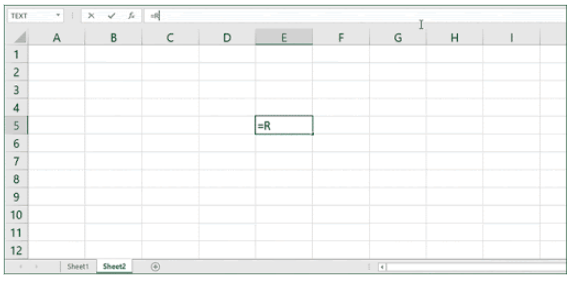4. You can also use cells as references in the formula. For example, you can use the =ROUNDDOWN(C4, E6) formula to round down the value in C4 to the matter in E6.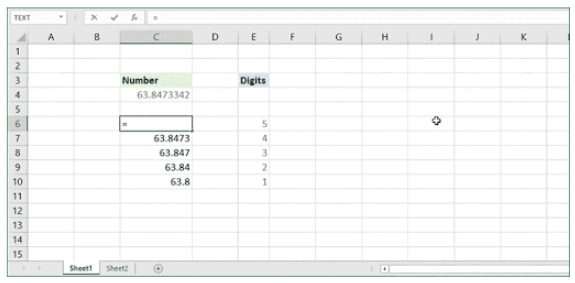## Excel Round-off Formula – Round down

In Excel, the round-off formula for rounding down will assist the user in rounding down the decimal value to the next lower whole number1.8630, for example, will be rounded up to the next most significant value of 1.8.

Now, calculate the round-off formula in Excel to round up the results by following the steps below. This example considers a student data collection in which you will attempt to round up the students’ percentage numbers.

Make a new column called “round-off % value” and rename it. To round-down down the first two decimal places, pick the first cell and type in the formula = ROUND(M2,2), as seen in the image below.

Choose the round-down option from the drop-down menu.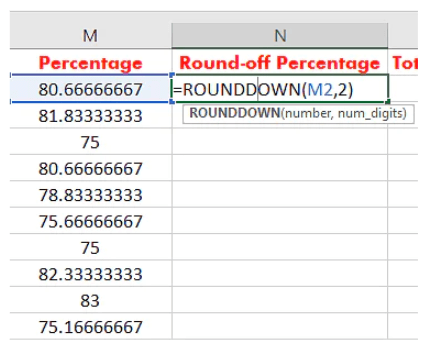As seen in the image below, press enter and drag the cell throughout the column to apply the formula to the entire column.### ExampleCopy and paste the example data from the table below into cell A1 of a new Excel worksheet. To see the results of formulas, select them, press F2, and then Enter. You can alter the column widths to see all the data if necessary.

#### In Excel, how to ROUND DOWN to one decimal place.

Click in cell C3, type the equal sign, and then type the’ ROUNDDOWN’ function. Then we’ll move on to an opening bracket, which will require two things.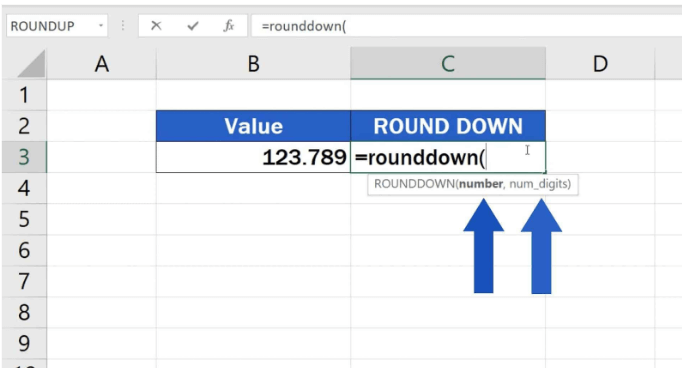The first will be the cell containing the amount we want to round down, so click on B3, then insert a comma before typing in the second value – the number indicating how many decimal places in the result.Type ‘1’, close the parenthesis and click ‘Enter’ to round down to one decimal place. Excel will display the result in C3, the value from B3 rounded to one decimal point.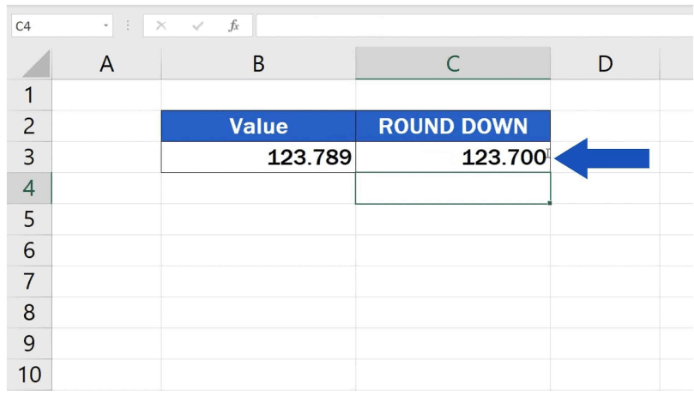In Excel, how to ROUND DOWN to two decimal places.

Use 2 in the function to round down to two decimal places, and the resulting integer will reduce down to two decimal places.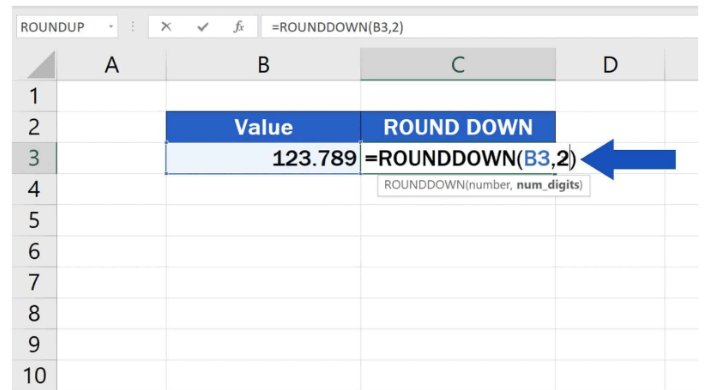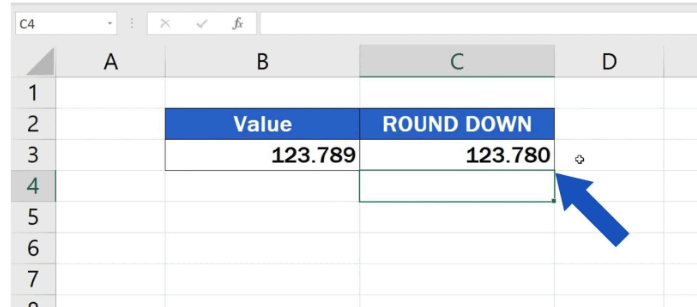1, 2, 3, and so on are all positive numbers that can be used to round down to any number of decimal places.

#### What is the top way to round up a value to make it a whole number?

Enter a zero in the function to round the value down to a whole number.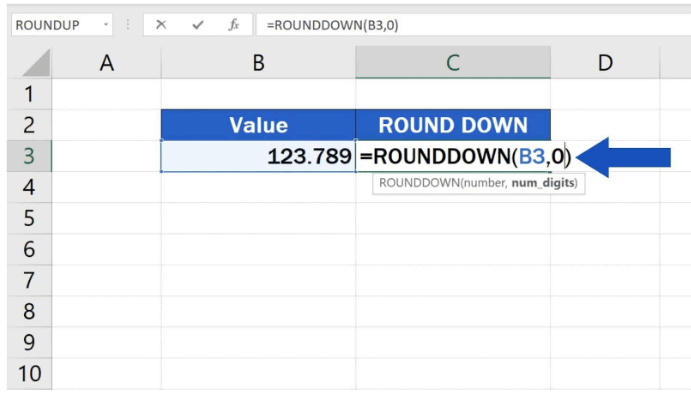And Excel will do precisely what you want – it will round down the original amount to a whole number, as shown below.#### How to round a number to closest the tens or hundreds

Users must use negative numbers in the formula to round a number down to tens or hundreds, which implies shifting to the left of the decimal point; therefore, include the minus sign with the number in parentheses.

I’ll give you a handful of instances.

Enter ‘-1’ into the formula to round the number down to the nearest tenth, and here’s the result!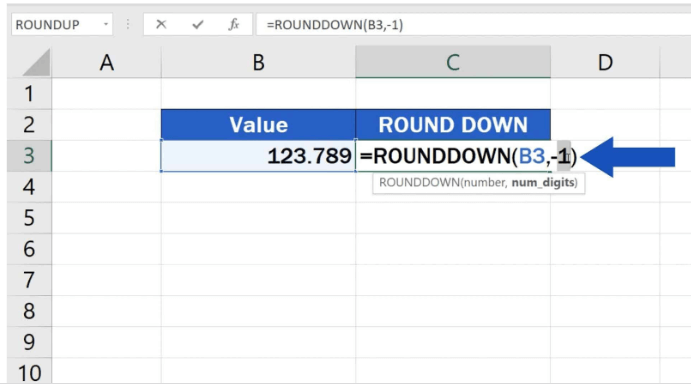If you wish to round a number down to the nearest hundredth, type ‘-2’, and Excel will do the math.

That’s how it’s done! This function can be used to round any number to the nearest tenth.

## Parting Shot

We hope this article has supported your understanding of utilizing Excel’s ROUNDDOWN function to round down figures quickly. This understanding may help you do numerous computations more easily and quickly when working with spreadsheets that need mathematics.

#### Follow us on our Social Media!

Shopping cart0
There are no products in the cart!
Continue shopping
0

Need Help?